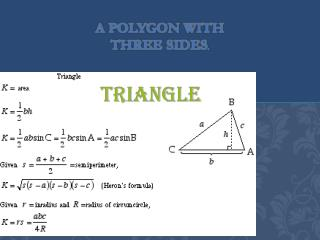DownloadDownload PresentationA polygon with three sides .

# A polygon with three sides .

Télécharger la présentation## A polygon with three sides .

- - - - - - - - - - - - - - - - - - - - - - - - - - - E N D - - - - - - - - - - - - - - - - - - - - - - - - - - -
##### Presentation Transcript

1. A polygon with three sides.

2. rectangle A box shape on a plane. Formally, a rectangle is a quadrilateral with four congruent angles (all 90°).

3. parallelogram • The perimeter of a parallelogram: • To find the perimeter of a parallelogram, just add up all the lengths of the sides: • Perimeter = a + b + a + b • = 2a + 2b • The area of a parallelogram: • To find the area of a parallelogram, just multiply the base length (b) times the height (h): • Area = b x h

4. Circles • Circle • The locus of all points that are a fixed distance from a given point. • AB = Diameter • Must pass through the center of the circle. The Diameter is equal to twice the radius. • OC = Radius • The radius is a line segment that begin from the center and touches any point on the circle. • ED = Chord • The chord joins any two points on a circle. • FG = Tangent • A line with one point common to the circle. • EHD = Arc • Only the portion of the circle that is between two points on the circle. • ADB = Semicircle • Also considered to be an arc that is exactly half of the circle. • OCB = Sector • The area between two radii and the arc. • COB = Central Angle • Formed by two radii

5. Irregular shapes • A regular octagon is a closed figure with sides of the same length and internal angles of the same size. It has eight lines of reflective symmetry and rotational symmetry of order 8. The internal angle at each vertex of a regular octagon is 135° and the sum of all the internal angles is 1080° (as for any octagon). The area of a regular octagon of side length a is given by • In terms of R (circumradius), the area is • In terms of r (in radius), the area is